# SBI CLERK PRELIMS – APTITUDE – 12

Dear Aspirants,

The SBI CLERK Prelims Exam 2018 is one of the important exam and we know you would be searching for the correct planner to devise your strategy. Since the exam pattern is changed , you would have been puzzled about how to study for the exam and make your preparations in the right way. Don’t fret and prepare as you wish but go in the right direction.

The Preliminary Examination of the SBI CLERK 2018 will happen in March/April , so you have to gear up your preparations in the right way to score more in the exams. So attend Daily Quizzes which will enrich your preparations and study and learn new topics which will help you for the exams.

Our Team will be providing the Daily Quizzes on various topics which were based on the previous exams and new pattern questions. This will help you to crack the exam and to achieve your goal. So attend and take the best result which could guide you to find the key of your dreams.SBI Clerk Prelims 2018 Study Planner Day – 13

Section : Quantitative Aptitude

Topic : Quadratic Equation – 2

Time: 20 Minutes

Directions.1-5): Solve the two given quantities and find out the relationship between them:

1.

Quantity 1: Area of square having its perimeter is 2 times of circumference of circle having 21mtrs as its radius.

Quantity 2: Area of a rectangle whose length is 150% of its breadth and which perimeter is 270mtrs.

a) Quantity 1 > Quantity 2

b) Quantity 1 < Quantity 2

c) Quantity 1 ≥ Quantity 2

d) Quantity 1 ≤ Quantity 2

e) Quantity 1 = Quantity 2 or Relationship can’t be established.

2.

Quantity 1 = 75x7÷(y3z2)

Quantity 2 = (23y4x3) ÷ z5

X = 1; Y = 2; Z =3

a) Quantity 1 > Quantity 2

b) Quantity 1 < Quantity 2

c) Quantity 1 ≥ Quantity 2

d) Quantity 1 ≤ Quantity 2

e) Quantity 1 = Quantity 2 or Relationship can’t be established.

3.

Quantity 1: Perimeter of a Square having its side is equal to hypotenuse of a right-angled triangle having its sides 8mtrs and 6mtrs respectively.

Quantity 2: Perimeter of a Rhombus having its diagonals d1 and d2 are 12mtr and 16mtr respectively.

a) Quantity 1 > Quantity 2

b) Quantity 1 < Quantity 2

c) Quantity 1 ≥ Quantity 2

d) Quantity 1 ≤ Quantity 2

e) Quantity 1 = Quantity 2 or Relationship can’t be established.

4.

Quantity 1: The value of “a” from 8a2+6a+10=15

Quantity 2: The value of “b” from 12b2+9b = 7.5

a) Quantity 1 > Quantity 2

b) Quantity 1 < Quantity 2

c) Quantity 1 ≥ Quantity 2

d) Quantity 1 ≤ Quantity 2

e) Quantity 1 = Quantity 2 or Relationship can’t be established.

5.

Quantity 1: The value of X from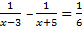Quantity 2: The value of X from x3+ y3+ z3– 3xyz =0, if the value of y = 6 and z = -10.

a) Quantity 1 > Quantity 2

b) Quantity 1 < Quantity 2

c) Quantity 1 ≥ Quantity 2

d) Quantity 1 ≤ Quantity 2

e) Quantity 1 = Quantity 2 or Relationship can’t be established.

D.6-10): In each of these questions, two equations (I) and (II) are given. You have to solve both the equations and give answer:

6.

I:16x2+14x+3=0

II:8y2+10y+3=0

a) If X > Y

b) If X < Y

c) If X ≥ Y

d) If Y ≤ Y

e) If X = Y (or) Relation cannot be established between ‘X’ and ‘Y’

7.

I: 8x+4 -3x+18=4-12x+9x+32-2x

II: 18y-12+13+4y+6+12y+21-27y+8=0

a) If X > Y

b) If X < Y

c) If X ≥ Y

d) If Y ≤ Y

e) If X = Y (or) Relation cannot be established between ‘X’ and ‘Y’

8.

I:7x2+11x-6=0

II:4x2-11x+6=0

a) If X > Y

b) If X < Y

c) If X ≥ Y

d) If Y ≤ Y

e) If X = Y (or) Relation cannot be established between ‘X’ and ‘Y’

9.

I: 7x+12y =24

II: 3x+7y= 1

a) If X > Y

b) If X < Y

c) If X ≥ Y

d) If Y ≤ Y

e) If X = Y (or) Relation cannot be established between ‘X’ and ‘Y’

10.

I: 144x2+96x-48=0

II: 169y2-234y-208=0

a) If X > Y

b) If X < Y

c) If X ≥ Y

d) If Y ≤ Y

e) If X = Y (or) Relation cannot be established between ‘X’ and ‘Y’

D.11-15): In each of these questions, two equations I and II are given. You have to solve both the equation and give answer:

11.

I. x2+22x+120=0

II.y2-20y-300=0

a) I < II

b) I ≥ II

c) I ≤ II

d) I > II

e) I = II (or) Relationship cannot be established

12.

I.x2+20x+51=0

II.y2-15y+56=0

a) I < II

b) I ≥ II

c) I ≤ II

d) I > II

e) I = II (or) Relationship cannot be established

13.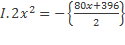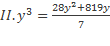a) I < II

b) I ≥ II

c) I ≤ II

d) I > II

e) I = II (or) Relationship cannot be established

14 .

a) I < II

b) I ≥ II

c) I ≤ II

d) I > II

e) I = II (or) Relationship cannot be established

15.

I.5x2+2x-51=0

II.y2+3y-54=0

a) I < II

b) I ≥ II

c) I ≤ II

d) I > II

e) I = II (or) Relationship cannot be established

D.16-20): In each of these questions, two equations I and II are given. You have to solve both the equation and give answer:

16.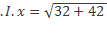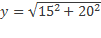a) I < II

b) I ≥ II

c) I ≤ II

d) I > II

e) I = II (or) Relationship cannot be established

17.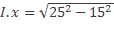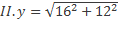I. x2-7x+12=0

II.y2+17y+72=0

a) I < II

b) I ≥ II

c) I ≤ II

d) I > II

e) I = II (or) Relationship cannot be established

18.

I.2x2+13x+21=0

II.3y2+11y+10=0

a) I < II

b) I ≥ II

c) I ≤ II

d) I > II

e) I = II (or) Relationship cannot be established

19.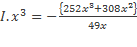II.y4=256

a) I < II

b) I ≥ II

c) I ≤ II

d) I > II

e) I = II (or) Relationship cannot be established

20.

I. 12x2-13x+3=0

II.6y2+13y+7=0

a) I < II

b) I ≥ II

c) I ≤ II

d) I > II

e) I = II (or) Relationship cannot be established

Solutions for the Above Questions :

1. (b)

2. (b)

3. (e)

4. (e)

5. (e)

6. (c)

7. (a)

8. (b)

9. (a)

10. (e)

11. (c)

12. (a)

13. (c)

14. (a)

15. (e)

16. (e)

17. (d)

18. (a)

19. (e)

20. (d)

Aspirants can also check the other topics from the SBI CLERK STUDY PLANNER from the link given below. DO bookmark the given link as all the posts will be updated in that page and aspirants can check the daily updates from this page or from the Homepage of Bankersdaily.

# SBI CLERK PRELIMS EXAM 2018 – STUDY PLANNER

Check the official notification of the SBI CLERK EXAM 2018 from the below provided links. Also the notification will enlighten you about the exam patterns and will answer various questions regarding the SBI CLERK EXAM 2018.